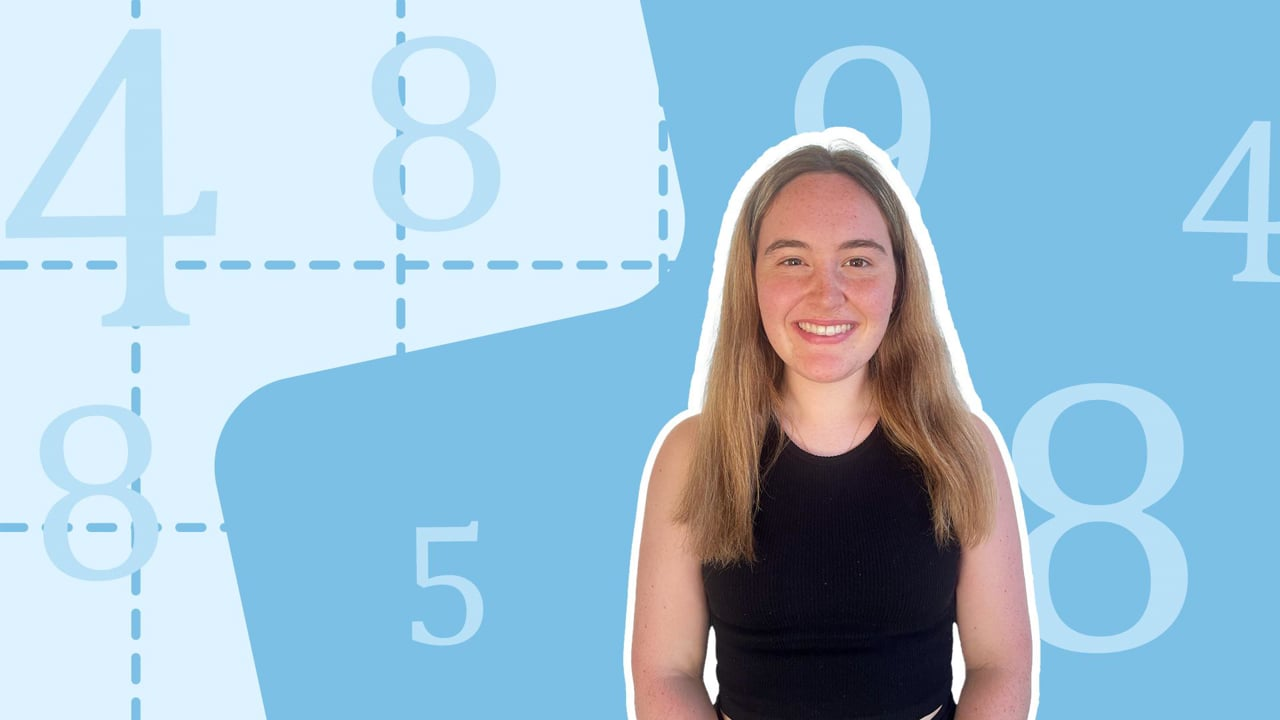Chapter overviewMaths

Number and place value

Multiplication and division

Fractions

Measurement

Geometry - properties of shapes

Geometry - position and direction

Statistics

Maths

# Multiplying a 4-digit number by a 1 or 2-digit number0%

Summary

# Multiplying a 4-digit number by a 1 or 2-digit number

## In a nutshell

Multiplying four-digit numbers by one- and two-digit numbers require short and long multiplication, as they are too long to be worked out mentally.

## Written multiplication methods

The long and short multiplication methods are written methods where columns are used to set out multiplication calculations.

### Multiplying a four-digit number by a one-digit number

When multiplying a four-digit number by a one-digit number, short multiplication is used. The calculation is set out as usual and multiplication by the number happens from right to left in columns.

##### Example 1

What is $1352\times6$?

Set out the calculation.

$\begin{array}{cccc}&\text{Th} & \text{H} & \text{T} & \text{O} \\& 1& 3 & 5 & 2 \\\times & & && 6 \\ \hline&&&& \\ \hline&&&&\end{array}$​​

Multiply each digit of the four-digit number by six.

$2\times6=12\\5\times6=30\\3\times6=18\\1\times6=1$​​

Place each answer in the correct column, carrying and adding where necessary.

$\begin{array}{cccc}&\text{Th} & \text{H} & \text{T} & \text{O} \\& 1& 3 & 5 & 2 \\\times & & && 6 \\ \hline&8&1&1& 2\\ \hline&^2&^3&^1&\end{array}$​​

$1352\times6=\underline{8112}$​​

### Multiplying a four-digit number by a two-digit number

Multiplying by a two-digit number is almost the same - the only difference is that the two digit number has to be partitioned into tens and units. This method is known as long multiplication.

#### PROCEDURE

 1 Set out the calculation:Write your numbers one below the other, in columns where tens and ones line up.Draw a multiplication symbol on the left. 2 Partition the two-digit number into tens and units and multiply each separately. 3 Adjust zeros accordingly. 4 Add the answers together.

##### Example 2

What is $1784\times27$?

Set out the calculation.

$\begin{array}{ccccc}&\text{Tth}&\text{Th} & \text{H} & \text{T} & \text{O} \\& &1 & 7& 8& 4 \\\times & & & &2& 7 \\ \hline& &&&& \\+& &&&& \\ \hline& &&&& \end{array}$​​

Partition $27$ into tens and ones.

$27=20+7$

First multiply by the ones.

$\begin{array}{ccccc}&\text{Tth}&\text{Th} & \text{H} & \text{T} & \text{O} \\& &1 & 7& 8& 4 \\\times & & & &2& \underline7 \\ \hline& ^11&^52&^54&^28&8 \\+& &&&& \\ \hline& &&&& \end{array}$​​

Then multiply by the tens.

Treat $20$ as $2$, then add a zero in the first column.

$\begin{array}{ccccc}&\text{Tth}&\text{Th} & \text{H} & \text{T} & \text{O} \\& &1 & 7& 8& 4 \\\times & & & &\underline2& 7 \\ \hline& ^11&^52&^54&^28&8 \\+& &&&&0 \\ \hline& &&&& \end{array}$​​

Continue multiplying by $2$

$\begin{array}{ccccc}&\text{Tth}&\text{Th} & \text{H} & \text{T} & \text{O} \\& &1 & 7& 8& 4 \\\times & & & &\underline2& 7 \\ \hline& ^11&^52&^54&^28&8 \\+& ^13&^15&6&8&0 \\ \hline& &&&& \end{array}$​​

$\begin{array}{ccccc}&\text{Tth}&\text{Th} & \text{H} & \text{T} & \text{O} \\& &1 & 7& 8& 4 \\\times & & & &\underline2& 7 \\ \hline& ^11&^52&^54&^28&8 \\+& ^13&^15&6&8&0 \\ \hline& 4&^18&^11&6&8 \end{array}$​​

​​

FAQs

• Question: How do I multiply a four-digit number by a one-digit number?

Answer: When multiplying a four-digit number by a one-digit number, short multiplication is used. The calculation is set out as usual and multiplication by the number happens from right to left in columns.

• Question: How do I multiply a four-digit number by a two-digit number?

Answer: To multiply a four-digit number by a two-digit number, use long multiplication. The steps are as follows: 1. Set out the calculation: - Write your numbers one below the other, in columns where tens and ones line up. - Draw a multiplication symbol on the left. 2. Partition the two-digit number into tens and units and multiply each separately. 3. Adjust zeros accordingly. 4. Add the answers together.

Theory

Exercises